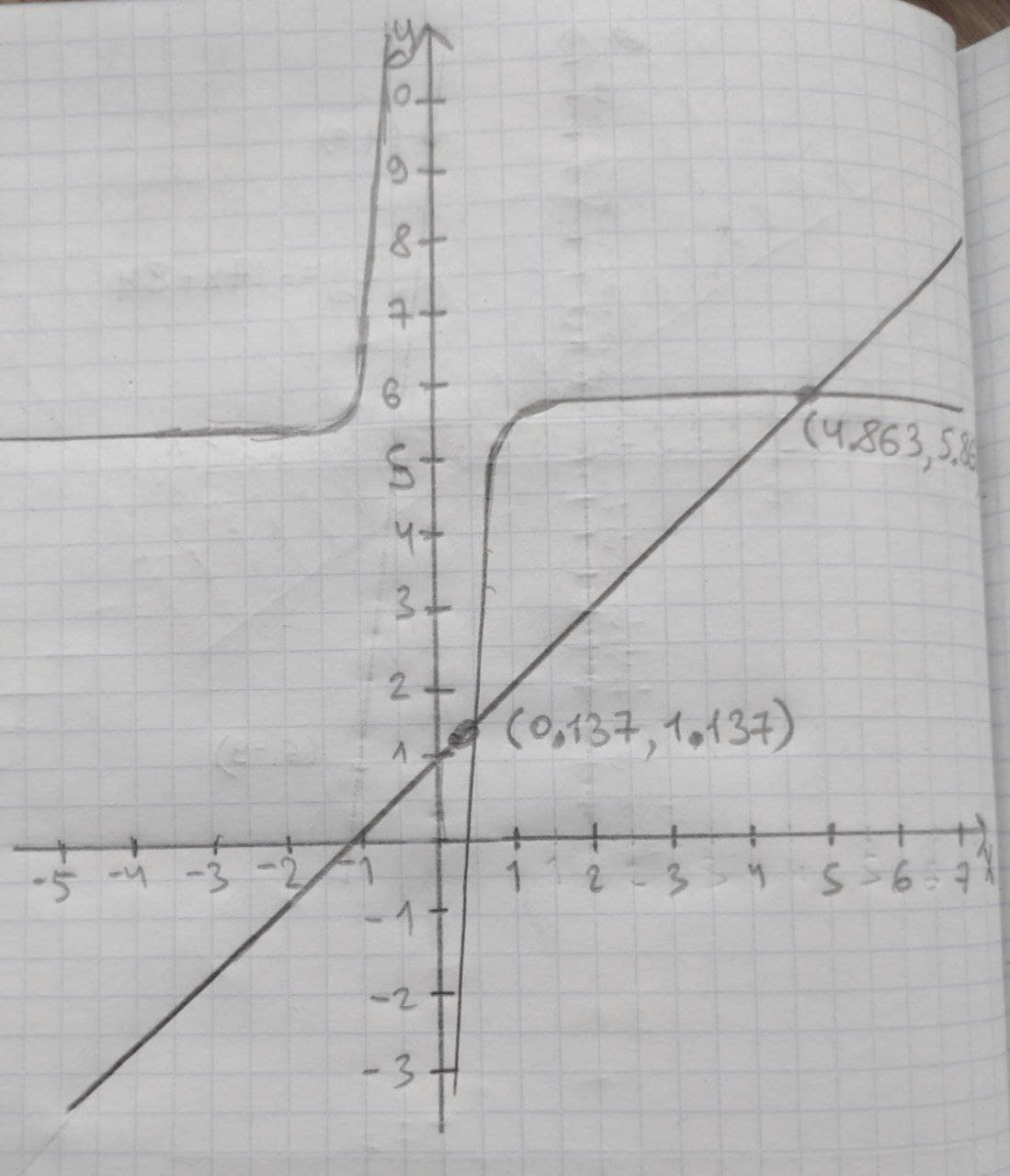# Graphing systems of linear equations find the solution for the following equations by graphing. Y= 6- 2/(3x) Y= x + 1hexacordoK 2021-01-31 Answered
Graphing systems of linear equations
find the solution for the following equations by graphing.
$Y=6-\frac{2}{3x}$
$Y=x+1$
You can still ask an expert for help

• Questions are typically answered in as fast as 30 minutes

Solve your problem for the price of one coffee

• Math expert for every subject
• Pay only if we can solve itodgovoreh
Graph the given two equations and find the intersection points as follows.Thus, the obtained solutions by graphing is,
(0.137, 1.137) and (4.863, 5.863)